# Divisors

Find all divisors of number 493. How many are them?

Correct result:

n =  4

#### Solution: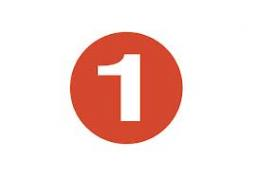We would be pleased if you find an error in the word problem, spelling mistakes, or inaccuracies and send it to us. Thank you!Tips to related online calculators
Do you want to perform natural numbers division - find the quotient and remainder?

## Next similar math problems:

• Percentages52 is what percent of 93?
• EquationsSolve following system of equations: 6(x+7)+4(y-5)=12 2(x+y)-3(-2x+4y)=-44
• Screws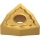The box contains 8 iron, 6 brass, and 4 titanium screws. What is the probability that the randomly selected screw will not be brass?
• Study results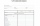There are 7 students in the class with excellent results, 6 praiseworthy, 5 good, 4 sufficient and 3 insufficient students. What is the probability that it will be a good student when summoned?
• The ballThe ball was discounted by 10 percent and then again by 30 percent. How many percent of the original price is now?
• Price increase 2xIf two consecutive times we increase the price of the product by 20%, how many % is higher final price than the original?
• Two numbersWe have two numbers. Their sum is 140. One-fifth of the first number is equal to half the second number. Determine those unknown numbers.
• Divisible by 5How many three-digit odd numbers divisible by 5, which are in place ten's number 3?
• Aircraft modelsHow many car models did the collector own after the collector's market? The collector of car and aircraft models owned 3 times more car models than aircraft. He sold 7 car models and 9 aircraft models on the stock exchange and bought 12 car models and 4 a
• Drawing from a hatWhen drawing numbers from a hat from 1 to 35, we select random given numbers. What is the probability that the drawn numbers will be divisible by 8 and 2?
• Two numbersThe difference between the two numbers is 74. If we divide a larger number by a smaller one, we get a quotient 7 and the rest of 2. Determine both numbers.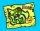There are 4 roads from city A to city B. There are 5 roads from city B to city C. How many different routes can we come from city A to city C via city B?Pavel has a collection of toy cars. He wanted to regroup them. But in the division of three, four, six, and eight, he was always one left. Only when he formed groups of seven, he divided everyone. How many toy cars have in the collection?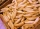Jano and Michael ate pagans. Jano ate three more than Michael. The product of their counts (numbers) is 180. How many pagans did each of them eat?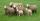Shepherd tending the sheep. Tourists asked him how much they have. The shepherd said, "there are fewer than 500. If I them lined up in 4-row 3 remain. If in 5-row 4 remain. If in 6-row 5 remain. But I can form 7-row." How many sheep have herdsman?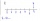Find four-digit numbers where all the digits are different. For numbers, the sum of the third and fourth digits is twice the sum of the first two digits, and the sum of the first and fourth digits is equal to the sum of the second and third digits. The di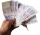How many different ways can the cashier payout € 310 if he uses only 50 and 20 euro banknotes? Find all solutions.